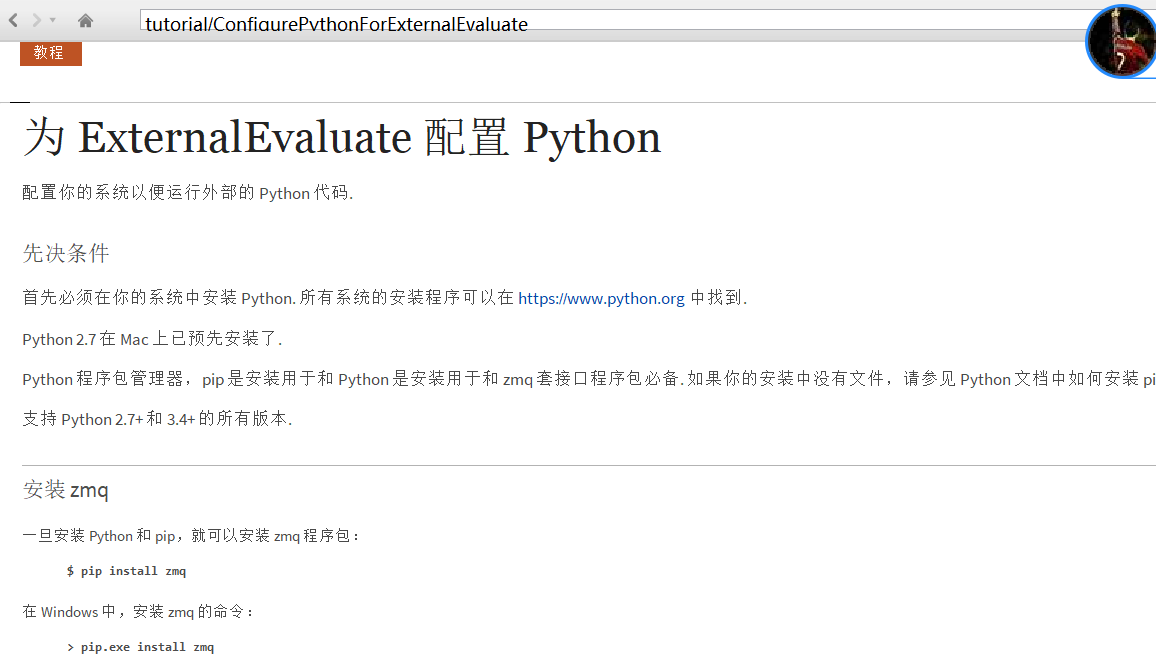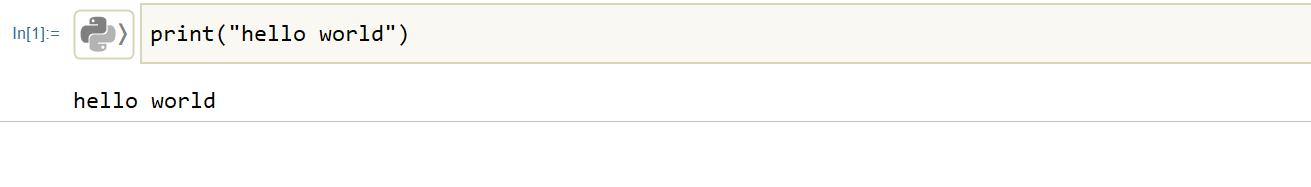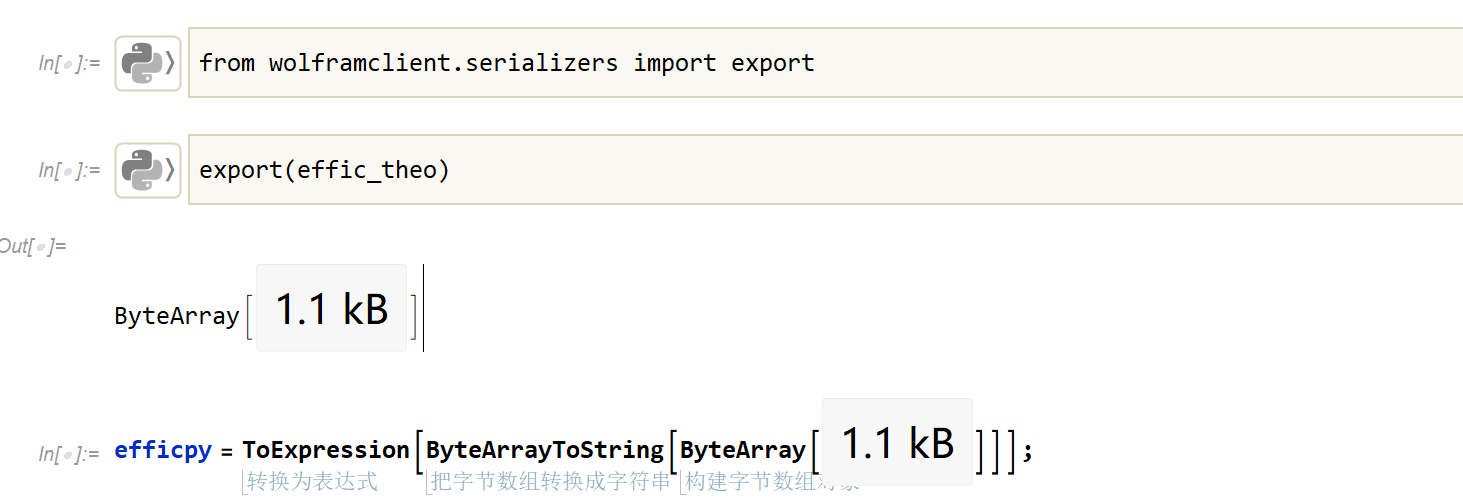# Mathematica

## 前言

​ 现如今Python很火，因为其入门简单，功能强大。在科学计算领域中也占有一席之地。本人作为量子光学领域的理论研究生，平时仿真软件(COMSOL,Lumerical)用的很多，MATLAB,Python以及Mathematica也用得勤。

​ 最后来说Mathematica，以前一直以为是个计算器，忽视了其作用，疫情期间花时间了解之后，知晓其功能之强大，也对Mathematica的创始人顶礼膜拜。Mathematica设计的初衷就是想做一个全能型选手，其在各个领域都有涉及，特别是符号计算，其编程的思路以及写程序的模式一旦适应，写起来真的很顺手。

## 为什么不直接用Mathematica而用Python？

​ Mathematica的符号计算让很多计算就像写公式一样简单，也许也正因为如此，Mathematica没有对应完善的量子信息包来处理数据。近日也有人和Wolfram介绍量子计算的Package,感兴趣的可以看这个讨论

Quantum Computing in Wolfram Language

Quantum Mathematica

## 为什么不用JupyterNotebook?

Jupyter Notebook确实很好用，但是Mathematica对我而言更好用, 就喜欢边写公式边计算个积分，算出个结果马上绘制个曲线，想看哪个pdF、图片、代码、仿真文件，直接通过Mathematica调用系统默认软件打开，Mathematica一个笔记本可以把一切都给你满足。而且其输入公式的逻辑比Latex好，所写即所见，我觉得用Mathematica推导比我用纸笔高效。Jupyter Notebook用Latex输入再公式很长的时候，修改起来贼麻烦。

Mathematica支持折叠，支持不同笔记本的互相引用，用过就知道有多好！

## 如何在Mathematica简单使用Python?

### 在Python安装库：pyzmq### 直接从Mathematica的“外部运行框”里面运行Python代码## 如何在Mathematica使用Python的函数、数据、图片?

### 如何让Python绘制的图片内置于Mathematica的Notebook界面内

How to display images inside Mathematica with matplotlib

### 如何在Mathematica中使用Python的数组Mathematica小技巧

### 如何在Python中使用Mathematica的数组

（更新于2021年12月17日）

https://community.wolfram.com/groups/-/m/t/2343086

![[Attachment/MMA模板.nb]]